[Introduction]  [Signal arithmetic]  [Signals and noise]   [Smoothing]   [Differentiation]  [Peak Sharpening]  [Harmonic analysis]   [Fourier convolution]  [Fourier deconvolution]  [Fourier filter]   [Peak area measurement]  [Linear Least Squares]  [Multicomponent Spectroscopy]  [Iterative Curve Fitting]  [Hyperlinear quantitative absorption spectrophotometry] [Appendix and Case Studies]  [Peak Finding and Measurement]  [iPeak]   [iSignal]  [Peak Fitters]   [iFilter]  [iPower]  [List of downloadable software]  [Interactive tools]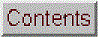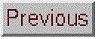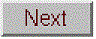## Curve fitting A: Linear Least Squares

[Basics]  [Reliability]  [Transforming non-linear relationships]  [Fitting peaks]  [Math details]  [Spreadsheets]  [LINEST function]  [Matlab]

The objective of curve fitting is to find the parameters of a mathematical model that describes a set of (usually noisy) data in a way that minimizes the difference between the model and the data. The most common approach is the "linear least squares" method, also called "polynomial least squares", a well-known mathematical procedure for finding the coefficients of polynomial equations that are a "best fit" to a set of X,Y data. A polynomial equation expresses the dependent variable Y as a weighted sum of a series of single-valued functions of the independent variable X, most commonly as a straight line (Y = a + bX, where a is the intercept and b is the slope), or a quadratic (Y = a + bX + cX2), or a cubic (Y = a + bX + cX2 + dX3), or higher-order polynomial. Those coefficients (a, b, c, etc) can be used to predict values of Y for each X. In all these cases, Y is a linear function of the parameters a, b, c, and/or d. This is why we call it a "linear" least-squares fit, not because the plot of X vs Y is linear. Only for the first-order polynomial Y = a + bX is the plot of X vs Y linear. And if the model can not be described by a weighted sum of a series of single-valued functions, then a different, more computationally laborious, "non-linear" least-squares method may be used, which will be discussed in a later section.

“Best fit” simply means that the differences between the actual measured Y values and the Y values predicted by the model equation are minimized. It does not mean a "perfect" fit; in most cases, a least-squares best fit does not go through all the points in the data set. Above all, a least-squares fit must conform to the selected model - for example, a straight line or a quadratic parabola - and there will almost always be some data points that do not fall exactly on the best-fit line, either because of random error in the data or because the model is not capable of describing the data exactly. It's not correct to say "fit data to ..." a straight line or to some other model; it's actually the other way around: you are fitting a model to the data. The data are not being modified in any way; it is the model that is being adjusted to fit the data.

Least-squares best fits can be calculated by some hand-held calculators, spreadsheets, and dedicated computer programs (see Math Details below). Although it is possible to estimate the best-fit straight line by visual estimation and a straightedge, the least-square method is more objective and easier to automate. (If you were to give a plot of X,Y data to five different people and ask them to estimate the best-fit line visually, you'd get five slightly different answers, but if you gave the data set to five different computer programs, you'd get the exact same answer every time).

Here's a very simple example: the historical prices of different sizes of SD memory cards advertised in the February 19, 2012, issue of the New York Times.  (Yes, I know, the prices are much lower now, but these were really the prices back in 2012).

 Memory Capacity in GBytes Price in US dollars 2 \$9.99 4 \$10.99 8 \$19.99 16 \$29.99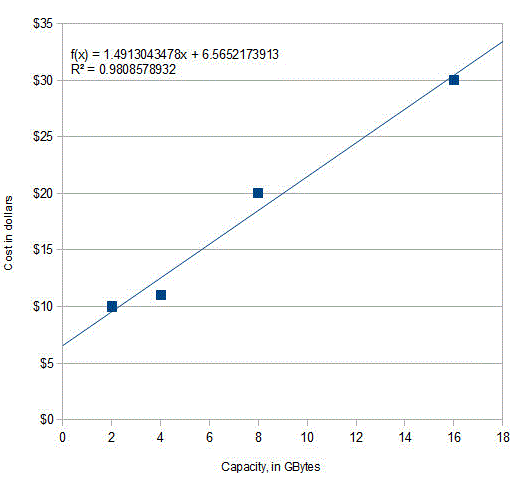What's the relationship between memory capacity and cost? Of course, we expect that the larger-capacity cards should cost more than the smaller-capacity ones, and if we plot cost vs capacity (graph on the right), we can see a rough straight-line relationship. A least-squares algorithm can compute the values of a (intercept) and b (slope) of the straight line that is a "best fit" to the data points. Using a linear least-squares calculation, where X = capacity and Y = cost, the straight-line mathematical equation that most simply describes these data (rounding to the nearest penny) is:

Cost = \$6.56 + Capacity * \$1.49

So, \$1.49 is the slope and \$6.56 is the intercept.  (The equation is plotted as the solid line that passes among the data points in the figure). Basically, this is saying that the cost of a memory card consists of a fixed cost of \$6.56 plus \$1.49 for each Gbyte of capacity. How can we interpret this? The \$6.56 represents the costs that are the same regardless of the memory capacity: a reasonable guess is that it includes things like packaging (the different cards are the same physical size and are packaged the same way), shipping, marketing, advertising, and retail shop shelf space. The \$1.49 (actually 1.49 dollars/Gbyte) represents the increasing retail price of the larger chips inside the larger capacity cards, which have more value for the consumer and also probably cost more to make because they use more silicon, are more complex, and have a higher chip-testing rejection rate in the production line. So in this case the slope and intercept have real physical and economic meanings.

What can we do with this information?  First, we can see how closely the actual prices conform to this equation: pretty well but not perfectly. The line of the equation passes among the data points but does not go exactly through each one. That's because actual retail prices are also influenced by several factors that are unpredictable and random: local competition, supply, demand, and even rounding to the nearest "neat" number; all those factors constitute the "noise" in these data.  The least squares procedure also calculates R2, called the coefficient of determination or the correlation coefficient, which is an indicator of the "goodness of fit". R2 is exactly 1.0000 when the fit is perfect, less than that when the fit is imperfect. The closer to 1.0000 the better. An R2 value of 0.99 means a fairly good fit; 0.999 is a very good fit.

The second way we can use these data is to predict the likely prices of other card capacities, if they were available, by putting in the memory capacity into the equation and evaluating the cost. For example, a 12 Gbyte card would be expected to cost \$24.44 according to this model. And a 32 Gbyte card would be predicted to cost \$54.29, but that would be predicting beyond the range of the available data - it's called “extrapolation”- and it's very risky because you don't really know what other factors may influence the data beyond the last data point. (You could also solve the equation for capacity as a function of cost and use it to predict how much capacity could be expected to be bought for a given amount of money, if such a product were available).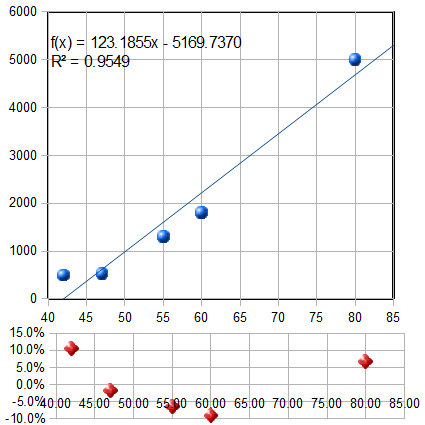Here's another related example: the historical prices of standard high definition (not UHD) flat-screen LCD TVs as a function of screen size, as advertised on the Web in the Spring of 2012. The prices of five selected models, similar except for screen size, are plotted against the screen size in inches in the figure on the left, and are fit to a first-order (straight-line) model. As for the previous example, the fit is not perfect. The equation of the best-fit model is shown at the top of the graph, along with the R2 value (0.9549) indicating that the fit is not very good. And you can see from the best-fit line that a 40 inch set would be predicted to have a negative cost! They would pay you to take these sets?  I don't think so. Clearly something is wrong here.

The goodness of fit is shown even more clearly in the little graph at the bottom of the figure, with the red dots. This shows the "residuals", the differences between each data point and the least-squares fit at that point. You can see that the deviations from zero are fairly large (±10%), but more important, they are not completely random; they form a clearly visible U-shaped curve. This is a tip-off that the straight-line model we have used here may not be ideal and that we might get a better fit with another model. (Or it might be just chance: the first and last points might be higher than expected because those were unusually expensive TVs for those sizes. How would you really know unless your data collection was very careful?).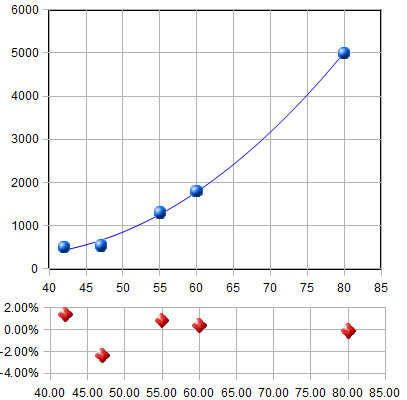Least-squares calculations can fit not only straight-line data, but any set of data that can be described by a polynomial, for example a second-order (quadratic) equation (Y = a + bX + cX2).  Applying a second-order fit to these data, we get the graph on the right. Now the R2 value is higher, 0.9985, indicating that the fit is better (but again not perfect), and also the residuals (the red dots at the bottom) are smaller and more random. This shouldn't really be a surprise, because the size of a TV screen is always quoted as the length of the diagonal, from one corner of the screen to its opposite corner, but the quantity of material, the difficulty of manufacture, the weight, and the power supply requirements of the screen should all scale with the screen area. Area is proportional to the square of the linear measure, so the inclusion of an X2 term in the model is quite reasonable in this case. With this fit, the 40 inch set would be predicted to cost under \$500, which is more sensible than the linear fit. (The actual interpretation of the meaning of the best-fit coefficients a, b, and c is, however, impossible unless we know much more about the manufacture and marketing of TVs). The least-squares procedure allows us to model the data with a more-or-less simple polynomial equation. The point here is that a quadratic model is justified not just because it fits the data better, but in this case because it is expected in principal on the basis of the relationship between length and area. (Incidentally, as you might expect, prices have dropped considerably since 2012; in 2015, a 65" flat-screen HDTV set was available at Costco for under \$900).

In general, fitting any set of data with a higher order polynomial, like a quadratic, cubic or higher, will reduce the fitting error and make the R2 values closer to 1.000, because a higher order model has more variable coefficients to adjust to the data. For example, we could fit the SD card price data to a quadratic, but there is no reason to do so and the fit would only be slightly better. The danger is that you could be "fitting the noise", that is, adjusting to the random noise in that particular data set, whereas another measurement with different random noise might give markedly different results. In fact, if you use a polynomial order that is one less that the number of data points, the fit will be perfect and R2=1.000. For example, the SD card data have only 4 data points, and if you fit those data to a 3rd order (cubic) polynomial, you'll get a mathematically perfect fit, but one that makes no sense in the real world (the price turns back down above x=14 Gbytes). It's really meaningless and misleading - any 4-point data would have fit a cubic model perfectly, even pure random noise. The only justification for using a higher order polynomial is if you have reason to believe that there is a consistent non-linearity in the data set, as in the TV price example above.

###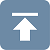A plot of the residuals for the calibration data (right) raises a question. Except for the 6th data point (at a concen­tration of 0.6), the other points seem to form a rough U-shaped curve, indicating that a quadratic equation might be a better model for those points than a straight line. Can we reject the 6th point as being an “outlier”, perhaps caused by a mistake in preparing that solution standard or in reading the instrument for that point? Discarding that point would improve the quality of fit (R2=0.992 instead of 0.986) especially if a quadratic fit were used (R2=0.998). The only way to know for sure is to repeat that standard solution preparation and calibration and see if that U shape persists in the residuals. Many instruments do give a very linear calibration response, while others may show a slightly non-linear response under some circumstances (for example). But in fact, the calibration data used for this particular example were computer-generated to be perfectly linear, with normally-distributed random numbers added to simulate noise. So actually that 6th point is really not an outlier and the underlying data are not really curved, but you would not know that in a real application. It would have been a mistake to discard that 6th point and use a quadratic fit in this case. Moral: don't throw out data points just because they seem a little off, unless you have good reason, and don't use higher-order polynomial fits just to get better fits if the instrument is known to give linear response under those circumstances. Even perfectly normally-distributed random errors can occasionally give individual deviations that are quite far from the average and might tempt you into thinking that they are outliers. Don't be fooled. (Full disclosure: I obtained the above example by “cherry-picking” from among dozens of randomly generated data sets, in order to find one that, although actually random, seemed to have an outlier).

Solving the calibration equation for concentration. Once the calibration curve is established, it can be used to determine the concentrations of unknown samples that are measured on the same instrument, for example by solving the equation for concentration as a function of instrument reading. The result for the linear case is that the concentration of the sample Cx is given by Cx = (Sx - intercept)/slope, where Sx is the signal given by the sample solution, and "slope" and "intercept" are the results of the least-squares fit. If a quadratic fit is used, then you must use the more complex "quadratic equation" to solve for concentration, but the problem of solving the calibration equation for concentration becomes forbiddingly complex for higher order polynomial fits. (The concentration and the instrument readings can be recorded in any convenient units, as long as the same units are used for calibration and for the measurement of unknowns).

### Reliability of  curve fitting resultsHow reliable are the slope, intercept and other polynomial coefficients obtained from least-squares calculations on experimental data? The single most important factor is the appropriateness of the model chosen; it's critical that the model (e.g. linear, quadratic, gaussian, etc) be a good match to the actual underlying shape of the data. You can do that either by choosing a model based on the known and expected behavior of that system (like using a linear calibration model for an instrument that is known to give linear response under those conditions) or by choosing a model that always gives randomly-scattered residuals that do not exhibit a regular shape. But even with a perfect model, the least-squares procedure applied to repetitive sets of measurements will not give the same results every time because of random error (noise) in the data. If you were to repeat the entire set of measurements many times and do least-squares calculations on each data set, the standard deviations of the coefficients would vary directly with the standard deviation of the noise and inversely with the square root of the number of data points in each fit, all else being equal. The problem, obviously, is that it is not always possible to repeat the entire set of measurements many times. You may have only one set of measurements, and each experiment may be very expensive to repeat. So, it would be great if we had a short-cut method that would let us predict the standard deviations of the coefficients from a single measurement of the signal, without actually repeating the measurements.

Here I will describe three general ways to predict the standard deviations of the polynomial coefficients: algebraic propagation of errors, Monte Carlo simulation, and the bootstrap sampling method.

Algebraic Propagation of errors.The classical way is based on the rules for mathematical error propagation. The propagation of errors of the entire curve-fitting method can be described in closed-form algebra by breaking down the method into a series of simple differences, sums, products, and ratios, and applying the rules for error propagation to each step. The result of this procedure for a first-order (straight line) least-squares fit are shown in the last three lines of the set of equations in Math Details, below. Essentially, these equations make use of the deviations from the least-squares line (the "residuals") to estimate the standard deviations of the slope and intercept, based on the assumption that the noise in that single data set is random and is representative of the noise that would be obtained upon repeated measurements. Because these predictions are based only on a single data set, they are good only insofar as that data set is typical of others that might be obtained in repeated measurements. If your random errors happen to be small when you acquire your data set, you'll get a deceptively good-looking fit, but then your estimates of the standard deviation of the slope and intercept will be too low, on average. If your random errors happen to be large in that data set, you'll get a deceptively bad-looking fit, but then your estimates of the standard deviation will be too high, on average. This problem becomes worse when the number of data points is small. This is not to say that it is not worth the trouble to calculate the predicted standard deviations of slope and intercept, but keep in mind that these predictions are accurate only if the number of data points is large (and only if the noise is random and normally distributed). Beware: if the deviations from linearity in your data set are systematic and not random - for example, if try to fit a straight line to a smooth curved data set - then the estimates the standard deviations of the slope and intercept by these last two equations will be too high, because they assume the deviations are caused by random noise that varies from measurement to measurement, whereas in fact a smooth curved data set without random noise will give the same slope and intercept from measurement to measurement.

In the application to analytical calibration, the concentration of the sample Cx is given by Cx = (Sx - intercept)/slope, where Sx is the signal given by the sample solution. The uncertainty of all three terms contribute to the uncertainty of Cx. The standard deviation of Cx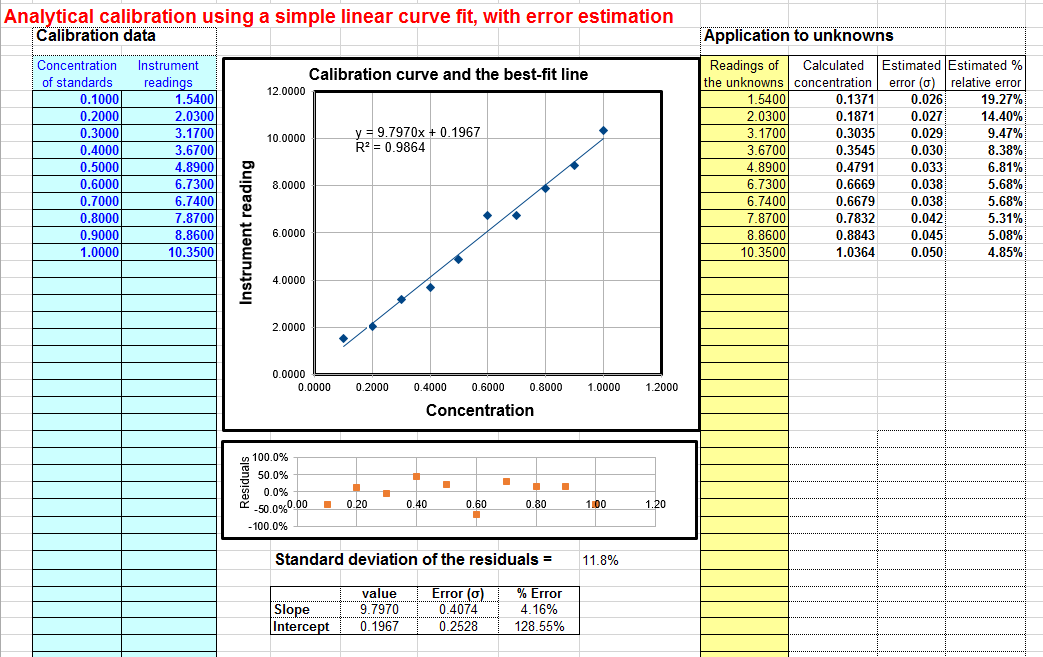can be estimated from the standard deviations of slope, intercept, and Sx using the rules for mathematical error propagation. But the problem is that, in analytical chemistry, the labor and cost of preparing and running large numbers of standards solution often limits the number of standards to a rather small set, by statistical standards, so these estimates of standard deviation are often fairly rough. A spreadsheet that performs these error-propagation calculations for your own first-order (linear) analytical calibration data can be downloaded from http://terpconnect.umd.edu/~toh/models/CalibrationLinear.xls). For example, the linear calibration example just given in the previous section, where the "true" value of the slope was 10 and the intercept was zero, this spreadsheet (whose screen shot shown on the right) predicts that the slope is 9.8 with a standard deviation 0.407 (4.2%) and that the intercept is 0.197 with a standard deviation 0.25 (128%), both well within two standard deviations of the true values. This spreadsheet also performs the propagation of error calculations for the calculated concentrations of each unknown in the last two columns on the right. In the example in this figure, the instrument readings of the standards are taken as the unknowns, showing that the predicted percent concentration errors range from about 5% to 19% of the true values of those standards. (Note that the standard deviation of the concentration is greater at high concentrations than the standard deviation of the slope, and considerably greater at low concentrations because of the greater influence of the uncertainly in the intercept). For a further discussion and some examples, see "The Calibration Curve Method with Linear Curve Fit". The downloadable Matlab/Octave plotit.m function uses the algebraic method to compute the standard deviations of least-squares coefficients for any polynomial order.

Monte Carlo simulation.The second way of estimating the standard deviations of the least-squares coefficients is to perform a random-number simulation (a type of Monte Carlo simulation). This requires that you know (by previous measurements) the average standard deviation of the random noise in the data. Using a computer, you construct a model of your data over the normal range of X and Y values (e.g. Y = intercept + slope*X + noise, where noise is the n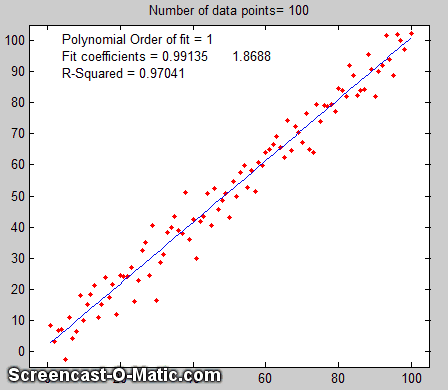oise in the data), compute the slope and intercept of each simulated noisy data set, then repeat that process many times (usually a few thousand) with different sets of random noise, and finally compute the standard deviation of all the resulting slopes and intercepts. This is ordinarily done with normally-distributed random noise (e.g. the RANDN function that many programming languages have). These random number generators produce "white" noise, but other noise colors can be derived. If the model is good and the noise in the data is well-characterized in terms of frequency distribution and signal amplitude dependence, the results will be a very good estimate of the expected standard deviations of the least-squares coefficients. (If the noise is not constant, but rather varies with the X or Y values, or if the noise is not white or is not normally distributed, then that behavior must be included in the simulation). An animated example is shown on the right, for the case of a 100-point straight line data set with slope=1, intercept=0, and standard deviation of the added noise equal to 5% of the maximum value of y. For each repeated set of simulated data, the fit coefficients (least-squares measured slope and intercept) are slightly different because of the noise.

Obviously this method involves programming a computer to compute the model and is not so convenient as evaluating a simple algebraic expression. But there are two important advantages to this method: (1) is has great generality; it can be applied to curve fitting methods that are too complicated for the classical closed-form algebraic propagation-of-error calculations, even iterative non-linear methods; and (2) its predictions are based on the average noise in the data, not the noise in just a single data set. For that reason, it gives more reliable estimations, particularly when the number of data points in each data set is small. Nevertheless, you can not always apply this method because you don't always know the average standard deviation of the random noise in the data. This type of computation is easily done in Matlab/Octave and in spreadsheets.

You can download a MatlabOctave script that compares the Monte Carlo simulation to the algebraic method above from http://terpconnect.umd.edu/~toh/spectrum/LinearFiMC.m. By running this script with different sizes of data sets ("NumPoints" in line 10), you can see that the standard deviation predicted by the algebraic method fluctuates a lot from run to run when NumPoints is small (e.g. 10), but the Monte Carlo predictions are much more steady. When NumPoints is large (e.g. 1000), both methods agree very well.

The Bootstrap.The third method is the "bootstrap" method, a procedure that involves choosing random sub-samples with replacement from a single data set and analyzing each sample the same way (e.g. by a least-squares fit). Every sample is returned to the data set after sampling, so that (a) a particular data point from the original data set could appear multiple times in a given sample, and (b) the number of elements in each bootstrap sub-sample equals the number of elements in the original data set. As a simple examp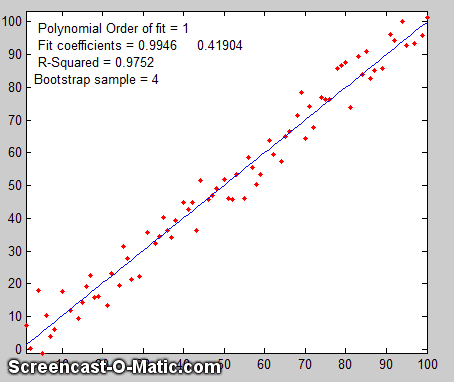le, consider a data set with 10 x,y pairs assigned the letters a through j. The original data set is represented as [a b c d e f g h i j], and some typical bootstrap sub-samples might be [a b b d e f f h i i] or [a a c c e f g g i j], each bootstrap sample containing the same number of data points, but with about a third of the data pairs skipped, a third duplicated, and a third left alone. (This is equivalent to weighting a third of the data pairs by a factor of 2, a third by 0, and a third unweighted). You would use a computer to generate hundreds or thousands of bootstrap samples like that and to apply the calculation procedure under investigation (in this case a linear least-squares) to each set.

If there were no noise in the data set, and if the model were properly chosen, then all the points in the original data set and in all the bootstrap sub-samples would fall exactly on the model line, with the result that the least-squares results would be the same for every sub-sample (click to see an animation).

But if there is noise in the data set, each set would give a slightly different result (e.g. the least-squares polynomial coefficients), because each sub-sample has a different subset of the random noise, as the animation on the right demonstrates.

The process is illustrated by the animation on the right, for the same 100-point straight-line data set used above. (You can see that the variation in the fit coefficients between sub-samples is the same as for the Monte Carlo simulation above). The greater the amount of random noise in the data set, the greater would be the range of results from sample in the bootstrap set. This enables you to estimate the uncertainty of the quantity you are estimating, just as in the Monte-Carlo method above. The difference is that the Monte-Carlo method is based on the assumption that the noise is known, random, and can be accurately simulated by a random-number generator on a computer, whereas the bootstrap method uses the actual noise in the data set at hand, like the algebraic method, except that it does not need an algebraic solution of error propagation. The bootstrap method thus shares its generality with the Monte Carlo approach, but is limited by the assumption that the noise in that (possibly small) single data set is representative of the noise that would be obtained upon repeated measurements. The bootstrap method cannot, however, correctly estimate the parameter errors resulting from poor model selection. The method is examined in detail in its extensive literature. This type of bootstrap computation is easily done in Matlab/Octave and can also be done (with greater difficulty) in spreadsheets.

Comparison of error prediction methods.The Matlab/Octave script TestLinearFit.m compares all three of these methods (Monte Carlo simulation, the algebraic method, and the bootstrap method) for a 100-point first-order linear least-squares fit. Each method is repeated on different data sets with the same average slope, intercept, and random noise, then the standard deviation (SD) of the slopes (SDslope) and intercepts (SDint)  were compiled and are tabulated below.

NumPoints = 100  SD of the Noise = 9.236 x-range = 30
Simulation    Algebraic equation  Bootstrap method
SDslope SDint    SDslope SDint      SDslope SDint
Mean SD:    0.1140  4.1158   0.1133  4.4821     0.1096  4.0203

(You can download this script from http://terpconnect.umd.edu/~toh/spectrum/TestLinearFit.m). On average, the mean standard deviation ("Mean SD") of the three methods agree very well, but the algebraic and bootstrap methods fluctuate more that the Monte Carlo simulation each time this script is run, because they are based on the noise in one single 100-point data set, whereas the Monte Carlo simulation reports the average of many data sets. Of course, the algebraic method is simpler and faster to compute than the other methods. However, an algebraic propagation of errors solution is not always possible to obtain, whereas the Monte Carlo and bootstrap methods do not depend on an algebraic solution and can be applied readily to more complicated curve-fitting situations, such as non-linear iterative least squares, as will be seen later.

Effect of the number of data points on least-squares fit precision.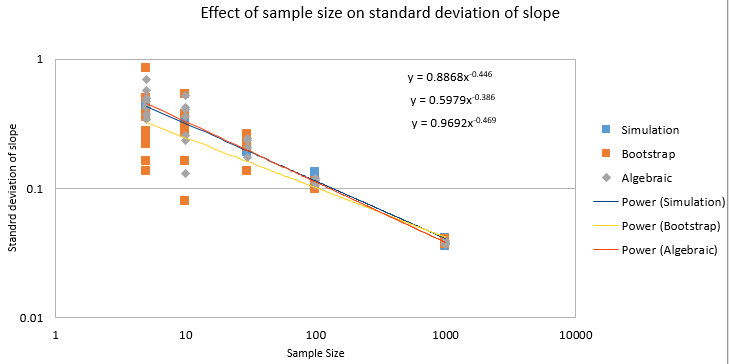The spreadsheets EffectOfSampleSize.ods or EffectOfSampleSize.xlsx, which collect the results of many runs of TestLinearFit.m with different numbers of data points ("NumPoints"), demonstrates that the standard deviation of the slope and the intercept decrease if the number of data points is increased; on average, the standard deviations are inversely proportional to the square root of the number of data points, which is consistent with the observation that the slope of a log-log plot is roughly 1/2.

These plots really dramatize the problem of small sample sizes, but this must be balanced against the cost of obtaining more data points. For example, in analytical chemistry calibration, a larger number of calibration points could be obtained either by preparing and measuring more standard solutions or by reading each of a smaller number of standards repeatedly. The former approach accounts for both the volumetric errors in preparing solutions and the random noise in the instrument readings, but the labor and cost of preparing and running large numbers of standard solutions, and safely disposing of them afterwards, is limiting. The latter approach is less expensive but is less reliable because it accounts only for the random noise in the instrument readings. Overall, it better to refine the laboratory techniques and instrument settings to minimize error that to attempts to compensate by taking lots of readings.

It's very important that the noisy signal not be smoothed before the least-squares calculations, because doing so will not improve the reliability of the least-squares results, but it will cause both the algebraic propagation-of-errors and the bootstrap calculations to seriously underestimate the standard deviation of the least-squares results. You can demonstrate using the most recent version of the script TestLinearFit.m by setting SmoothWidth in line 10 to something higher than 1, which will smooth the data before the least-squares calculations. This has no significant effect on the actual standard deviation as calculated by the Monte Carlo method, but it does significantly reduce the predicted standard deviation calculated by the algebraic propagation-of-errors and (especially) the bootstrap method. For similar reasons, if the noise is pink rather than white, the bootstrap error estimates will also be low.  Conversely, if the noise is blue, as occurs in processed signals that have been subjected to some sort of differentiation process or that have been deconvoluted from some blurring process, then the errors predicted by the algebraic propagation-of-errors and the bootstrap methods will be high. (You can prove this to yourself by running TestLinearFit.m with pink and blue noise modes selected in lines 23 and 24). Bottom line: error prediction works best for white noise.

### Transforming non-linear relationshipsIn some cases a fundamentally non-linear relationship can be transformed into a form that is amenable to polynomial curve fitting by means of a coordinate transformation (e.g. taking the log or the reciprocal of the data), and then least-squares method can be applied to the resulting linear equation. For example, the signal in the figure below is from a simulation of an exponential decay (X=time, Y=signal intensity) that has the mathematical form Y = a exp(bX), where a is the Y-value at X=0 and b is the decay constant. This is a fundamentally non-linear problem because Y is a non-linear function of the parameter b. However, by taking the natural log of both sides of the equation, we obtain ln(Y)=ln(a) + bX. In this equation, Y is a linear function of both parameters ln(a) and b, so it can be fit by the least squares method in order to estimate ln(a) and b, from which you get a by computing exp(ln(a)). In this particular example, the "true" values of the coefficients are a =1 and b = -0.9, but random noise has been added to each data point, with a standard deviation equal to 10% of the value of that data point, in order to simulate a typical experimental measurement in the laboratory. An estimate of the values of  ln(a) and b, given only the noisy data points, can be determined by least-squares curve fitting of ln(Y) vs X.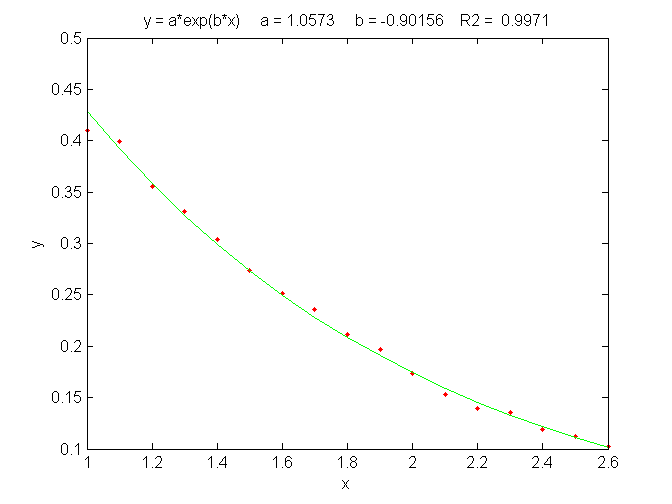An exponential least-squares fit (solid line) applied to a noisy data set (points) in order to estimate the decay constant, .

The best fit equation, shown by the green solid line in the figure, is Y =0.959 exp(- 0.905 X), that is, a = 0.959 and b = -0.905, which are reasonably close to the expected values of 1 and -0.9, respectively. Thus, even in the presence of substantial random noise (10% relative standard deviation), it is possible to get reasonable estimates of the parameters of the underlying equation (to within about 4%). The most important requirement is that the model be good, that is, that the equation selected for the model accurately describes the underlying behavior of the system (except for noise). Often that is the most difficult aspect, because the underlying models are not always known with certainty.  In Matlab and Octave, is fit can be performed in a single line of code: polyfit(x,log(y),1), which returns [b log(a)]. (In Matlab and Octave, "log" is the natural log, "log10" is the base-10 log).

Another example of the linearization of an exponential relationship is explored in in Appendix R: Signal and Noise in the Stock Market.

Other examples of non-linear relationships that can be linearized by coordinate transformation include the logarithmic (Y = a ln(bX)) and power (Y=aXb) relationships. Methods of this type used to be very common back in the days before computers, when fitting anything but a straight line was difficult. It is still used today to extend the range of functional relationships that can be handled by common linear least-squares routines available in spreadsheets and hand-held calculators. (The downloadable Matlab/Octave function trydatatrans.m tries eight different simple data transformations on any given x,y data set and fits the transformed data to a straight line or polynomial). Only a few non-linear relationships can be handled by simple data transformation, however. To fit any arbitrary custom function, you may have to resort to the iterative curve fitting method, which will be treated in Curve Fitting C.

Fitting Gaussian and Lorentzian peaks.An interesting example of the use of transformation to convert a non-linear relationship into a form that is amenable to polynomial curve fitting is the use of the natural log (ln) transformation to convert a positive Gaussian peak, which has the fundamental functional form exp(-x2), into a parabola of the form -x2, which can be fit with a second order polynomial (quadratic) function (y = a + bx + cx2). The equation for a Gaussian peak is y = h*exp(-((x-p)./(1/(2*sqrt(ln(2)))*w)) ^2)), where h is the peak height, p is the x-axis location of the peak maximum, w is the full width of the peak at half-maximum. The natural log of y can be shown to be log(h)-(4 p^2 log(2))/w^2+(8 p x log(2))/w^2-(4 x^2 log(2))/w^2, which is a quadratic form in the independent variable x because it is the sum of x^2, x, and constant terms. Expressing each of the peak parameters h, p, and w in terms of the three quadratic coefficients, a little algebra (courtesy of Wolfram Alpha) will show that all three parameters of the peak (height, maximum position, and width) can be calculated from the three quadratic coefficients a, b, and c; it's a classic "3 unknowns in 3 equations" problem. The peak height is given by exp(a-c*(b/(2*c))^2), the peak position by -b/(2*c), and the peak half-width by 2.35482/(sqrt(2)*sqrt(-c)). This is called "Caruana's Algorithm"; see Streamlining Digital Signal Processing: A "Tricks of the Trade" Guidebook, Richard G. Lyons, ed., page 298One advantage of this type of Gaussian curve fitting, as opposed to simple visual estimation, is illustrated in the figure on the left. The signal is a Gaussian peak with a true peak height of 100 units, a true peak position of 100 units, and a true half-width of 100 units, but it is sparsely sampled only every 31 units on the x-axis. The resulting data set, shown by the red points in the upper left, has only 6 data points on the peak itself.  If we were to take the maximum of those 6 points (the 3rd point from the left, with x=87, y=95) as the peak maximum, we'd get only a rough approximation to the true values of peak position (100) and height (100).  If we were to take the distance between the 2nd the 5th data points as the peak width, we'd get 3*31=93, compared to the true value of 100.

However, taking the natural log of the data (upper right) produces a parabola that can be fit with a quadratic least-squares fit (shown by the blue line in the lower left). From the three coefficients of the quadratic fit, we can calculate much more accurate values of the Gaussian peak parameters, shown at the bottom of the figure (height=100.93; position=99.11; width=99.25). The plot in the lower right shows the resulting Gaussian fit (in blue) displayed with the original data (red points). The accuracy of those peak parameters (about 1% in this example) is limited only by the noise in the data.

This figure was created in Matlab (or Octave), using this script. (The Matlab/Octave function gaussfit.m performs the calculation for an x,y data set. You can also download a spreadsheet that does the same calculation; it's available in OpenOffice Calc (Download link, Screen shot) and Excel formats).  Note: in order for this method to work properly, the data set must not contain any zeros or negative points; if the signal-to-noise ratio is very poor, it may be useful to skip those points or to pre-smooth the data slightly to reduce this problem. Moreover, the original Gaussian peak signal must be a single isolated peak with a zero baseline, that is, must tend to zero far from the peak center. In practice, this means that any non-zero baseline must be subtracted from the data set before applying this method. (A more general approach to fitting Gaussian peaks, which works for data sets with zeros and negative numbers and also for data with multiple overlapping peaks, is the non-linear iterative curve fitting method, which will be treated later).

A similar method can be derived for a Lorentzian peak,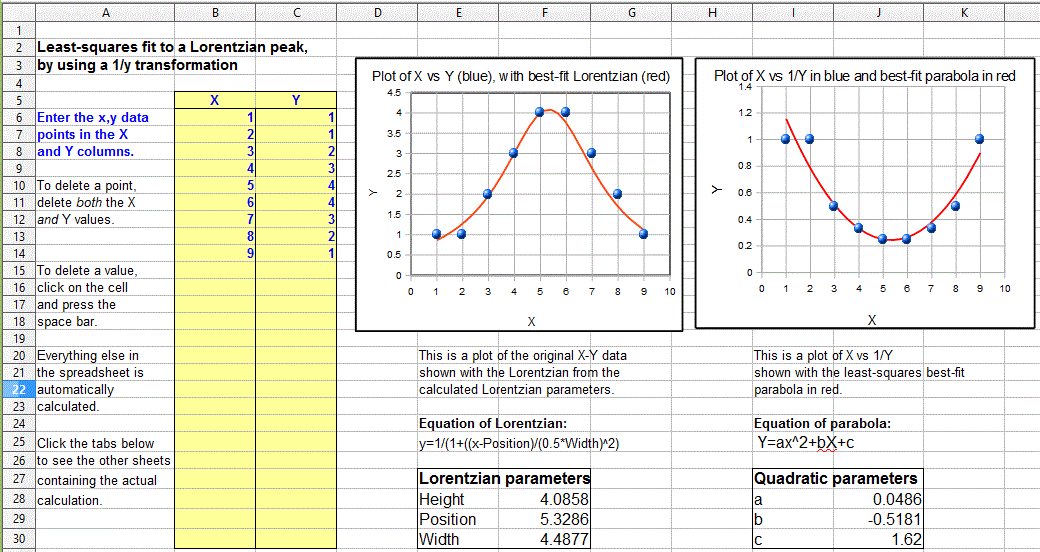which has the fundamental form y=h/(1+((x-p)/(0.5*w))^2), by fitting a quadratic to the reciprocal of y. As for the Gaussian peak, all three parameters of the peak (height h, maximum position p, and width w) can be calculated from the three quadratic coefficients a, b, and c of the quadratic fit: h=4*a/((4*a*c)-b^2), p= -b/(2*a), and w= sqrt(((4*a*c)-b^2)/a)/sqrt(a). Just as for the Gaussian case, the data set must not contain any zero or negative y values. The Matlab/Octave function lorentzfit.m performs the calculation for an x,y data set, and the Calc and Excel spreadsheets LorentzianLeastSquares.ods and LorentzianLeastSquares.xls perform the same calculation. (By the way, a quick way to test either of the above methods is to use this simple peak data set: x=5, 20, 35 and y=5, 10, 5, which has a height, position, and width equal to 10, 20, and 30, respectively, for a single isolated symmetrical peak of any shape, assuming a baseline of zero).

In order to apply the above methods to signals containing two or more Gaussian or Lorentzian peaks, it's necessary to locate all the peak maxima first, so that the proper groups of points centered on each peak can be processed with the algorithms just discussed. That is discussed in the section on Peak Finding and Measurement

But there is a downside to using coordinate transformation methods to convert non-linear relationships into simple polynomial form, and that is that the noise is also effected by the transformation, with the result that the propagation of error from the original data to the final results is often difficult to predict. For example, in the method just described for measuring the peak height, position, and width of Gaussian or Lorentzian peaks, the results depends not only on the amplitude of noise in the signal, but also on how many points across the peak are taken for fitting. In particular, as you take more points far from the peak center, where the y-values approach zero, the natural log of those points approaches negative infinity as y approaches zero. The result is that the noise of those low-magnitude points is unduly magnified and has a disproportional effect on the curve fitting. This runs counter the usual expectation that the quality of the parameters derived from curve fitting improves with the square root of the number of data points (CurveFittingC.html#Noise).  A reasonable compromise in this case is to take only the points in the top half of the peak, with Y-values down to one-half of the peak maximum.If you do that, the error propagation (predicted by a Monte Carlo simulation with constant normally-distributed random noise) shows that the relative standard deviations of the measured peak parameters are directly proportional to the noise in the data and inversely proportional to the square root of the number of data points (as expected), but that the proportionality constants differ:

relative standard deviation of the peak height = 1.73*noise/sqrt(N),

relative standard deviation of the peak position = noise/sqrt(N),

relative standard deviation of the peak width = 3.62*noise/sqrt(N),

where noise is the standard deviation of the noise in the data and N in the number of data points taken for the least-squares fit. You can see from these results that the measurement of peak position is most precise, followed by the peak height, with the peak width being the least precise. If one were to include points far from the peak maximum, where the signal-to-noise ratio is very low, the results would be poorer than predicted. These predictions depend on knowledge of the noise in the signal; if only a single sample of that noise is available for measurement, there is no guarantee that sample is a representative sample, especially if the total number of points in the measured signal is small; the standard deviation of small samples is notoriously variable. Moreover, these predictions are based on a simulation with constant normally-distributed white noise; had the actual noise varied with signal level or with x-axis value, or if the probability distribution had been something other than normal, those predictions would not necessarily have been accurate. In such cases the bootstrap method has the advantage that it samples the actual noise in the signal.

You can download the Matlab/Octave code for this Monte Carlo simulation from http://terpconnect.umd.edu/~toh/spectrum/GaussFitMC.m; view screen capture. A similar simulation (http://terpconnect.umd.edu/~toh/spectrum/GaussFitMC2.m, view screen capture) compares this method to fitting the entire Gaussian peak with the iterative method in Curve Fitting 3, finding that the precision of the results are only slightly better with the (slower) iterative method.

Note 2: In the curve fitting techniques described here and in the next two sections, there is no requirement that the x-axis interval between data points be uniform, as is the assumption in many of the other signal processing techniques previously covered.  Curve fitting algorithms typically accept a set of arbitrarily-spaced x-axis values and a corresponding set of y-axis values.

### Math details.The least-squares best fit for an x,y data set can be computed using only basic arithmetic.  Here are the relevant equations for computing the slope and intercept of the first-order best-fit equation, y = intercept + slope*x, as well as the predicted standard deviation of the slope and intercept, and the coefficient of determination, R2, which is an indicator of the "goodness of fit". (R2 is 1.0000 if the fit is perfect and less than that if the fit is imperfect).

 n = number of x,y data points    sumx = Σx    sumy = Σy    sumxy = Σx*y    sumx2 = Σx*x    meanx = sumx / n    meany = sumy / n    slope = (n*sumxy - sumx*sumy) / (n*sumx2 - sumx*sumx)    intercept = meany-(slope*meanx)    ssy = Σ(y-meany)^2    ssr = Σ(y-intercept-slope*x)^2    R2 = 1-(ssr/ssy) Standard deviation of the slope = SQRT(ssr/(n-2))*SQRT(n/(n*sumx2 - sumx*sumx)) Standard deviation of the intercept = SQRT(ssr/(n-2))*SQRT(sumx2/(n*sumx2 - sumx*sumx))

(In these equations, Σ represents summation; for example, Σx means the sum of all the x values, and Σx*y means the sum of all the x*y products, etc).

The last two lines predict the standard deviation of the slope and the intercept, based only on that data sample, assuming that the deviations from the line are random and normally distributed. These are estimates of the variability of slopes and intercepts you are likely to get if you repeated the data measurements over and over multiple times under the same conditions, assuming that the deviations from the straight line are due to random variability and not systematic error caused by non-linearity. If the deviations are random, they will be slightly different from time to time, causing the slope and intercept to vary from measurement to measurement, with a standard deviation predicted by these last two equations. However, if the deviations are caused by systematic non-linearity, they will be the same from from measurement to measurement, in which case the prediction of these last two equations will not be relevant, and you might be better off using a.polynomial fit such as a quadratic or cubic.

The reliability of these standard deviation estimates depends on assumption of random deviations and also on the number of data points in the curve fit; they improve with the square root of the number of points. A slightly more complex set of equations can be written to fit a second-order (quadratic or parabolic) equations to a set of data; instead of a slope and intercept, three coefficients are calculated, a, b, and c, representing the coefficients of the quadratic equation ax2+bx+c.

These calculations could be performed step-by-step by hand, with the aid of a calculator or a spreadsheet, with a program written in any programming language, such as a Matlab or Octave script.

Web sites: Wolfram Alpha includes some capabilities for least-squares regression analysis, including linear, polynomial, exponential, and logarithmic fits. Zunzun can curve fit and surface fit 2D and 3D data online with a rich set of error histograms, error plots, curve plots, surface plots, contour plots, VRML, auto-generated source code, and PDF file output. Statpages.org can perform a huge range of statistical calculations and tests, and there are several Web sites that specialize in plotting and data visualization that have curve-fitting capabilities, including most notably Tableau, Plotly and Plotter. Web sites such as these can be very handy when working from a smartphone, tablet, or a computer that does not have suitable computational software.

###Spreadsheets can perform the math described above easily; the spreadsheets pictured above (LeastSquares.xls and LeastSquares.odt for linear fits and (QuadraticLeastSquares.xls and QuadraticLeastSquares.ods for quadratic fits), utilize the expressions given above to compute and plot linear and quadratic (parabolic) least-squares fit, respectively. The advantage of spreadsheets is that they are highly customizable for your particular application and can be deployed on mobile devices such as tablets or smartphones. For straight-line fits, you can use the convenient built-in functions slope and intercept.

The LINEST function. Modern spreadsheets also have built-in facilities for computing polynomial least-squares curve fits of any order. For example, the LINEST function in both Excel and OpenOffice Calc can be used to compute polynomial and other curvilinear least-squares fits. In addition to the best-fit polynomial coefficients, the LINEST function also calculates at the same time the standard error values, the determination coefficient (R2), the standard error value for the y estimate, the F statistic, the number of degrees of freedom, the regression sum of squares, and the residual sum of squares. A significant inconvenience of LINEST, compared to working out the math using the series of mathematical expressions described above, is that it is more difficult to adjust to a variable number of data points and to remove suspect data points or to change the order of the polynomial. LINEST is an array function, which means that when you enter the formula in one cell, multiple cells will be used for the output of the function. You can't edit a LINEST function just like any other spreadsheet function. To specify that LINEST is an array function, do the following. Highlight the entire formula, including the "=" sign. On the Macintosh, next, hold down the “apple” key and press "return." On the PC hold down the “Ctrl” and “Shift” keys and press “Enter.” Excel adds "{ }" brackets around the formula, to show that it is an array. Note that you cannot type in the "{ }" characters yourself; if you do Excel will treat the cell contents as characters and not a formula. Highlighting the full formula and typing the “apple” key or “Ctrl”+”Shift” and "return" is the only way to enter an array formula. This instruction sheet  from Colby College may help.
Note: If you are working with a template that uses the LINEST function, and you wish to change the number of data points, the easiest way to do that is to select the rows or columns containing the data, right-click on the row or column heading (1,2,3 or a,b,c, etc). and use the Insert or Delete in the right-click menu. If you do it that way, the LINEST function referring to those rows or columns will be adjusted automatically. That's easier than trying to edit the LINEST function directly. (If you are inserting rows or columns, you must drag-copy the formulas from the older rows or columns into the newly inserted empty ones). See CalibrationCubic5Points.xls for an example.

Application to analytical calibration and measurement.

###There is also another set of spreadsheets that perform Monte Carlo simulations of the calibration and measurement process using several widely-used analytical calibration methods, including first-order (straight line) and second order (curved line) least squares fits. Typical systematic and random errors in both signal and in volumetric measurements are included, for the purpose of demonstrating how non-linearity, interferences, and random errors combine to influence the final result (the so-called "propagation of errors").

For fitting peaks, GaussianLeastSquares.odt, is an OpenOffice spreadsheet that fits a quadratic function to the natural log of y(x) and computes the height, position, and width of the Gaussian that is a best fit to y(x).  There is also an Excel version (GaussianLeastSquares.xls). LorentzianLeastSquares.ods and LorentzianLeastSquares.xls fits a quadratic function to the reciprocal of y(x) and computes the height, position, and width of the Lorentzian that is a best fit to y(x). Note that for either of the peaks fits, the data may not contain zeros or negative points, and the baseline (value that y approaches far from the peak center) must be zero. See Fitting Peaks, above.
SPECTRUM, the freeware signal-processing application for Mac OS8, includes a simple least-squares curve fitting for linear (straight-line), polynomials of order 2 through 5, and exponential, logarithmic, and power relationships.

###Matlab and Octave have simple built-in functions for least-squares curve fitting: polyfit and polyval. For example, if you have a set of x,y data points in the vectors "x" and "y", then the coefficients for the least-squares fit are given by coef=polyfit(x,y,n), where "n" is the order of the polynomial fit: n = 1 for a straight-line fit, 2 for a quadratic (parabola) fit, etc. The polynomial coefficients 'coef" are given in decreasing powers of x. For a straight-line fit (n=1), coef(1) is the slope ("b") and coef(2) is the intercept ("a"). For a quadratic fit (n=2), coef(1) is the x2 term ("c"), coef(2) is the x term ("b") and coef(3) is the constant term ("a").

The fit equation can be evaluated using the function polyval, for example fity=polyval(coef,x). This works for any order of polynomial fit ("n"). You can plot the data and the fitted equation together using the plot function: plot(x,y,'ob',x,polyval(coef,x),'-r'), which plots the data as blue circles and the fitted equation as a red line. You can plot the residuals by writing plot(x,y-polyval(coef,x)).

When the number of data points is small, you might notice that the fitted curve is displayed as a series of straight-line segments, which can look ugly. You can get a smoother plot of the fitted equation, evaluated at more finely divided values of x, by defining xx=linspace(min(x),max(x)); and then using xx rather than x to evaluate and plot the fit: plot(x,y,'ob',xx,polyval(coef,xx),'-r').

[
coef,S] = polyfit(x,y,n) returns the polynomial coefficients coef and a structure 'S' used to obtain error estimates.
>> [coef,S]=polyfit(x,y,1)
coef =
1.4913    6.5552
S =
R: [2x2 double]
df: 2
normr: 2.2341
>> S.R
ans =
-18.4391   -1.6270
0   -1.1632
The vector of standard deviations of the coefficients can be computed from S by: sqrt(diag(inv(S.R)*inv(S.R')).*S.normr.^2./S.df)', in the same order as the coefficients.

Matrix Method. Alternatively, you may perform the polynomial least squares calculations for the row vectors x,y without using the Matlab/Octave built-in polyfit function by using the matrix method with the Matlab "/" symbol, meaning "right matrix divide".  The coefficients of a first order fit are given by y/[x;ones(size(y))] and a second order (quadratic) fit by y/[x.^2;x;ones(size(y))] . For higher-order polynomials, just add another row to the denominator matrix, for example a third-order fit would be y/[x.^3;x.^2;x;ones(size(y))] and so on. The coefficients are returned in the same order as polyfit, in decreasing powers of x (e,g, for a first-order fit, slope first (the x^1 term) then intercept (the x^0 term). Using the example of the first-order fit to the SD memory card prices:

>>x=[2 4 8 16];
>> y=[9.99 10.99 19.99 29.99];
>> polyfit(x,y,1)
ans =
1.4913    6.5552
>> y/[x;ones(size(y))]
ans =
1.4913    6.5552

which shows that the slope and intercept results for the polyfit function and for the matrix method are the same. (The slope and intercept results are the same, but the polyfit function has the advantage that it also can compute the error estimates with little extra affort, as described above).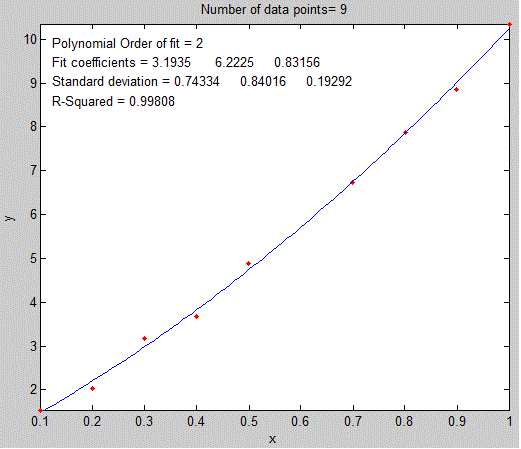The plotit.m function.

###The graph on the left (click to see a larger size) was generated by a simple downloadable Matlab/Octave function plotit(data) or plotit(data,polyorder), that uses all the techniques mentioned in the previous paragraph. It accepts 'data' in the form of a single vector, or a pair of vectors "x" and "y", or a 2xn or nx2 matrix with x in first row or column and y in the second, and plots the data points as red dots.

If the optional input argument "polyorder" is provided, plotit fits a polynomial of order "polyorder" to the data and plots the fit as a green line and displays the fit coefficients and the goodness-of-fit measure R2 in the upper left corner of the graph.

Here is a Matlab/Octave example of the use of plotit.m to perform the coordinate transformation described in a previous section to fit an exponential relationship, showing both the original exponential data and the transformed data with a linear fit in the figure(2) and figure(1) windows, respectively (click to download):

x=1:.1:2.6;
a=1;
b=-.9;
y = a.*exp(b.*x);
y=y+y.*.1.*rand(size(x));
figure(1)
[coeff,R2]=plotit(x,log(y),1);
ylabel('ln(y)');
title('Plot of x vs the natural log (ln) of y')
aa=exp(coeff(2));
bb=coeff(1);
yy= aa.*exp(bb.*x);
figure(2)
plot(x,y,'r.',x,yy,'g')
xlabel('x');
ylabel('y');
title(['y = a*exp(b*x)     a = ' num2str(aa)  '     b = ' num2str(bb)  '    R2 =  ' num2str(R2) ] ) ;

In version 5 or 6 the syntax of plotit can be  [coef, RSquared, StdDevs] = plotit(x,y,n). It returns the best-fit coefficients 'coeff', in decreasing powers of x, the standard deviations of those coefficients 'StdDevs' in the same order, and the R-squared value. To compute the relative standard deviations, just type StdDevs./coef. For example, the following script computes a straight line with 5 data points and a slope of 10, an intercept of zero, and noise equal to 1.0. then uses plotit.m to plot and fit the data to a first-order linear model (straight line) and compute the estimated standard deviation of the slope and intercept, if you run this over and over again, you will observe that the measured slope and intercept are almost always withing two standard deviations of 10 and zero respectively. Try it with different values of Noise.

NumPoints=5;
slope=10;
Noise=1;
x=round(10.*rand(size(1:NumPoints)));
y=slope*x+Noise.*randn(size(x));
[coef,RSquared,StdDevs]=plotit(x,y,1)

Comparing two data sets. Plotit can also be used to compare to two different dependent variable vectors (e.g. y1 and y2) if they share the same independent variables x, for example to determine the similarity of two different spectra measured over the same wavelengths as was done previously by simple division:

[coeff,R2]=plotit(y1,y2,1)

The closer the R2 value is to 1.000, the more similar they are. If y1 and y2 are two measurements of the same signal with different random noise, the plot will show a random scatter of points along a straight line with a slope of 1. If the y1 and y2 are
the same signal with different amplitudes, the slope of the line will equal their average ratio. If the data points are curved and loop around, the two y vectors are more different than the random noise.

In version 6 the syntax can be
optionally plotit(x,y,n,datastyle,fitstyle), where datastyle and fitstyle are optional strings specifying the line and symbol style and color, in standard Matlab convention. The strings, in single quotes, are made from one element from any or all the following 3 columns:
b   blue        .   point             -    solid
g   green       o   circle            :    dotted
r   red         x   x-mark            -.   dashdot
c   cyan        +   plus              --   dashed
m   magenta     *   star            (none) no line
y   yellow      s   square
k   black       d   diamond
w   white       v   triangle (down)
^   triangle (up)
<   triangle (left)
>   triangle (right)
p   pentagram
h   hexagram

For example,
plotit(x,y,3,'or','-g') plots the data as red circles and the fit as a green solid line (the default is red dots and a blue line, respectively).

You can use plotit.m to linearize and plot other nonlinear relationships, such as:
y = a exp(bx) : [coeff,R2]=plotit(x,log(y),1); a=exp(coeff(2)); b=coeff(1);
y = a ln(bx) : [coeff,R2]=plotit(log(x),y,1); a=coeff(1); b=log(coeff(2));
y=axb : [coeff,R2]=plotit(log(x),log(y),1); a=exp(coeff(2)); b=coeff(1);
y=start(1+rate)x:
[coeff,R2]=plotit(x,log(y),1); start=exp(coeff(2)); rate=exp(coeff(1))-1;
This last one is the expression for compound interest, which is used in Appendix R: Signal and Noise in the Stock Market.
Don't forget that in Matlab/Octave, "log" means natural log; the base-10 log is denoted by "log10".

Estimating the coefficient errors. The plotit function version 2 also has a built-in bootstrap routine that computes coefficient error estimates by the bootstrap method (Standard deviation STD and relative standard deviation RSD) and returns the results in the matrix "BootResults" (of size 5 x polyorder+1). You can change the number of bootstrap samples in line 101. The calculation is triggered by including a 4th output argument, e.g. [coef, RSquared, StdDevs, BootResults]= plotit(x,y,polyorder). This works for any polynomial order.  For example:

>> x=0:100;

>> y=100+(x*100)+100.*randn(size(x));
>> [FitResults, GOF, baseline, coeff, residual, xi, yi, BootResults] = plotit(x,y,1);

computes straight line with an intercept and slope of 100, plus random noise with a standard deviation of 100, then fits a straight line to that data and prints out a table of bootstrap error estimates, with the slope in the first column and the intercept in the second column:

Bootstrap Results

Mean:        100.359      88.01638
STD:         0.204564      15.4803
STD (IQR):   0.291484      20.5882
% RSD:       0.203832      17.5879
% RSD (IQR): 0.290441      23.3914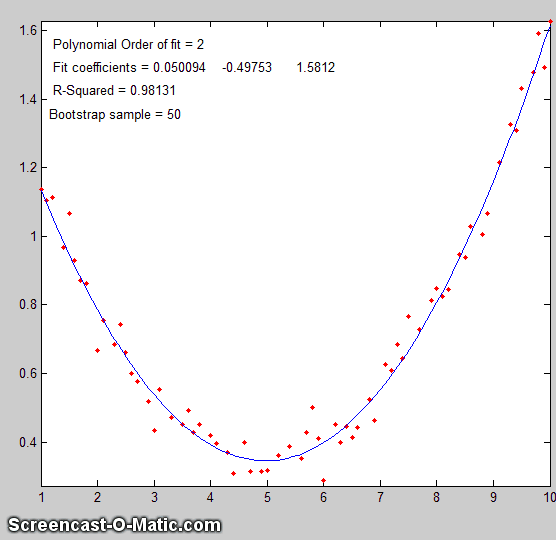The variation plotfita animates the bootstrap process for instructional purposes, as shown in the animation on the right a quadratic fit. You must include the output arguments, for example:
[coef, RSquared, BootResults]=plotfita([1 2 3 4 5 6],[1 3 4 3 2 1],2);

The variation logplotfit plots and fits log(x) vs log(y), for data that follows a power law relationship or that covers a very wide numerical range.

Finding the right polynomial order. My downloadable function trypoly(x,y) fits the data in x,y with a series of polynomials of degree 1 through length(x)-1 and returns the coefficients of determination (R2) of each fit as a vector, showing that, for any data, the coefficient of determination R2 approaches 1 as the polynomial order approaches length(x)-1. The variant trypolyplot(x,y) creates a bar graph.  Here's an example graphic

Finding the right data transformation. The related
function trydatatrans(x,y,polyorder) tries 8 different simple data transformations on the data x,y, fits the transformed data to a polynomial of order 'polyorder', displays results graphically in 3 x 3 array of small plots and returns the R2 values in a vector.

Fitting Gaussian and Lorentzian peaks.

###A simple user-defined Matlab/Octave function that fits a Gaussian function to an x,y signal is gaussfit.m, which implements the x vs ln(y) quadratic fitting method described above. It takes the form [Height,Position,Width]=gaussfit(x,y). For example,

>> x=50:150;y=100.*gaussian(x,100,100)+10.*randn(size(x));
>> [Height,Position,Width]=gaussfit(x,y)

returns [Height,Position,Width] clustered around 100,100,100. A similar function for Lorentzian peaks is lorentzfit.m,which takes the form

[Height,Position,Width]=lorentzfit(x,y).

An expanded variant of the gaussfit.m function is bootgaussfit.m, which does the same thing but also optionally plots the data and the fit and computes estimates of the random error in the height, width, and position of the fitted Gaussian function by the bootstrap sampling method.  For example:

>> x=50:150;y=100.*gaussian(x,100,100)+10.*randn(size(x));
>> [Height,Position,Width,BootResults]=bootgaussfit(x,y,1);

This does the same as the previous example but also displays error estimates in a table and returns the 3x5 matrix BootResults. Type "help bootgaussfit" for help.

Height     Position       Width
Bootstrap Mean: 100.84     101.325      98.341
Bootstrap STD:  1.3458     0.63091      2.0686
Bootstrap IQR:  1.7692     0.86874      2.9735
Percent RSD:    1.3346     0.62266      2.1035
Percent IQR:    1.7543     0.85737      3.0237

It's important that the noisy signal (x.y) not be smoothed if the bootstrap error predictions are to be accurate. Smoothing the data will cause the bootstrap method to seriously underestimate the precision of the results.

The gaussfit.m and lorentzfit.m functions are simple and easy but they do not work well with very noisy peaks or for overlapping peaks. As a demonstration, OverlappingPeaks.m is a demo script that shows how to use gaussfit.m to measure two overlapping partially gaussian peaks.   It requires careful selection of the optimum data regions around the top of each peak. Try changing the relative position and height of the second peak or adding noise (line 3) and see how it effects the accuracy. This function needs the gaussian.m, gaussfit.m, and peakfit.m functions in the path. The script also performs a measurement by the iterative method using peakfit.m, which is more accurate but takes about times longer to compute

The downloadable Matlab-only functions iSignal.m and ipf.m, whose principal functions are fitting peaks, also have a function for fitting polynomials of any order (Shift-o).

Recent versions of Matlab have a convenient tool for interactive manually-controlled (rather than programmed) polynomial curve fitting in the Figure window. Click for a video example: (external link to YouTube).

The Matlab Statistics Toolbox includes two types of bootstrap functions, "bootstrp" and "jackknife".  To open the reference page in Matlab's help browser, type "doc bootstrp" or "doc jackknife".###Last updated April, 2019. This page is part of "A Pragmatic Introduction to Signal Processing", created and maintained by Prof. Tom O'Haver , Department of Chemistry and Biochemistry, The University of Maryland at College Park. Comments, suggestions and questions should be directed to Prof. O'Haver at toh@umd.edu.
Unique visits since May 17, 2008: